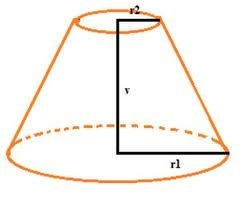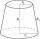# Truncated cone

Find the volume and surface area of the truncated cone if r1 = 12 cm, r2 = 5 cm and side s = 10 cm.

V =  1712.5734 cm3
S =  1064.9999 cm2

### Step-by-step explanation:Did you find an error or inaccuracy? Feel free to write us. Thank you!Tips to related online calculators
Tip: Our volume units converter will help you with the conversion of volume units.
Pythagorean theorem is the base for the right triangle calculator.

#### You need to know the following knowledge to solve this word math problem:

We encourage you to watch this tutorial video on this math problem:

## Related math problems and questions:

• Frustrum - volume, areaCalculate the surface and volume of the truncated cone, the radius of the smaller figure is 4 cm, the height of the cone is 4 cm and the side of the truncated cone is 5 cm.
• Truncated pyramidFind the volume and surface area of a regular quadrilateral truncated pyramid if base lengths a1 = 17 cm, a2 = 5 cm, height v = 8 cm.
• Truncated coneA truncated cone has bases with radiuses of 40 cm and 10 cm and a height of 25 cm. Calculate its surface area and volume.
• Truncated cone 5The height of a cone 7 cm and the length of side is 10 cm and the lower radius is 3cm. What could the possible answer for the upper radius of truncated cone?
• The cone - S,VCalculate the volume and surface area of the cone if its radius r = 6 cm and side s = 10 cm.
• Cone sideCalculate the volume and area of the cone whose height is 10 cm and the axial section of the cone has an angle of 30 degrees between height and the cone side.
• The surfaceThe surface of a truncated rotating cone with side s = 13 cm is S = 510π cm2. Find the radii of the bases when their difference in lengths is 10cm.
• Rotating coneFind the rotating cone's surface and volume if its side is 150 mm long and the circumference of the base is 43.96 cm.
• The truncatedThe truncated rotating cone has bases with radii r1 = 8 cm, r2 = 4 cm and height v = 5 cm. What is the volume of the cone from which the truncated cone originated?
• Lateral surface areaThe ratio of the area of the base of the rotary cone to its lateral surface area is 3: 5. Calculate the surface and volume of the cone, if its height v = 4 cm.
• Cone - sideFind the cone's surface area and volume if its height is 125 mm and the side length is 17 cm.
• Lamp coneCalculate the surface of a lampshade shaped of a rotary truncated cone with a base diameter of 32 cm and 12 cm and height of 24 cm.
• Truncated cone 3The surface of the truncated rotating cone S = 7697 meters square, the substructure diameter is 56m and 42m, find the height of the tang.
• Cone - from volume surface areaThe volume of the rotating cone is 1,018.87 dm3, and its height is 120 cm. What is the surface area of the cone?
• The diagram 2The diagram shows a cone with slant height 10.5cm. If the curved surface area of the cone is 115.5 cm2. Calculate correct to 3 significant figures: *Base Radius *Height *Volume of the cone
• Surface of the coneCalculate the surface of the cone if its height is 8 cm and the volume is 301.44 cm3.
• CalculateCalculate the cone's surface and volume that results from the rotation of the right triangle ABC with the squares 6 cm and 9 cm long around the shorter squeegee.